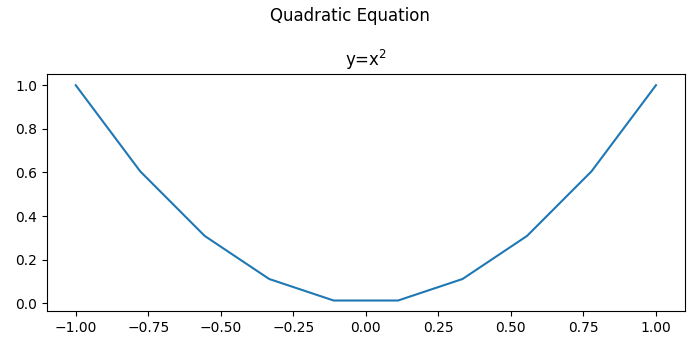# How to increase plt.title font size in Matplotlib?

To increase plt.title font size, we can initialize a variable fontsize and can use it in the title() method's argument.

## Steps

• Create x and y data points using numpy.

• Use subtitle() method to place the title at the center.

• Plot the data points, x and y.

• Set the title with a specified fontsize.

• To display the figure, use show() method.

## Example

import numpy as np
from matplotlib import pyplot as plt
plt.rcParams["figure.figsize"] = [7.00, 3.50]
plt.rcParams["figure.autolayout"] = True
x = np.linspace(-1, 1, 10)
y = x ** 2
fontsize = 12
plt.title("y=x$^{2}$", fontdict={'fontsize': fontsize})
plt.show()